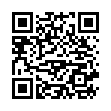# North Coast Synthesis Ltd.

## Cm4+2+♯1Choose audio sample set:
MSK 007 Leapfrog VCF (patch; see in online store)
MSK 009 Coiler VCF (patch; see in online store)

Equivalent chord symbols: Cm4+2+♭2, Cm4+2+♭9, E♭2+6+7+♯7, E♭2+6+7+♭1, D♭3+2+♯1+♯7, D♭3+2+♯1+♭1.

Notes in this chord: C, C♯, D, E♭, F. Integer notation: {0, 1, 2, 3, 5}.

Nearby chords (one less note): Cm4+2, E♭2+6+7, C2+♯1+♯2, D♭3+2+♯1, D♭3+♯1+♯7.

Nearby chords (one more note): D♭M9+♯1, F7+6+♯5, B♭+2+4+♯2, D♭M9♭5+♯1, C4+2+♯1+♯2, Cdim+2+4+♯1, Bdim+2+♯1+♯3.

Parallel chords (same structure, different root): Dm4+2+♯1, Em4+2+♯1, Fm4+2+♯1, Gm4+2+♯1, Am4+2+♯1, Bm4+2+♯1, D♭m4+2+♯1, E♭m4+2+♯1, G♭m4+2+♯1, A♭m4+2+♯1, B♭m4+2+♯1.

Experimental fretting charts for guitar standard EADGBE tuning (change tuning or instrument):